### 15.4.3.1 The Lie algebra

The notion of a Lie algebra is first established in general. Let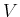be any vector space with coefficients in. In, the vectors can be added or multiplied by elements of; however, there is no way to multiply'' two vectors to obtain a third. The Lie algebra introduces a product operation to. The product is called a bracket or Lie bracket (considered here as a generalization of the previous Lie bracket) and is denoted by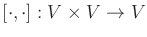.

To be a Lie algebra obtained from, the bracket must satisfy the following three axioms:

1. Bilinearity: For any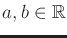and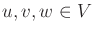,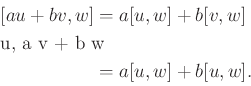(15.95)

2. Skew symmetry: For any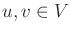,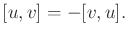(15.96)

This means that the bracket is anti-commutative.
3. Jacobi identity: For any,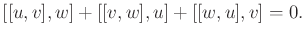(15.97)

Note that the bracket is not even associative.
Let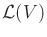denote the Lie algebra of. This is a vector space that includes all elements ofand any new elements that can be obtained via Lie bracket operations. The Lie algebraincludes every vector that can be obtained from any finite number of nested Lie bracket operations. Thus, describing a Lie algebra requires characterizing all vectors that are obtained under the algebraic closure of the bracket operation. Sinceis a vector space, this is accomplished by finding a basis of independent vectors of which all elements ofcan be expressed as a linear combination.

Example 15..14 (The Vector Cross Product)   Letbe the vector space over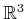that is used in vector calculus. The basis elements are often denoted as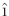,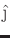, and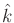. A bracket for this vector space is simply the cross product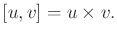(15.98)

It can be verified that the required axioms of a Lie bracket are satisfied.

One interesting property of the cross product that is exploited often in analytic geometry is that it produces a vector outside of the span ofand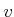. For example, let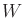be the two-dimensional subspace of vectors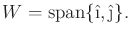(15.99)

The cross product always yields a vector that is a multiple of, which lies outside ofif the product is nonzero. This behavior is very similar to constructing vector fields that lie outside of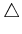using the Lie bracket in Section 15.4.2.Example 15..15 (Lie Algebra on Lie Groups)   Lie groups are the most important application of the Lie algebra concepts. Recall from Section 4.2.1 the notion of a matrix group. Important examples throughout this book have been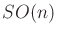and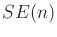. If interpreted as a smooth manifold, these matrix groups are examples of Lie groups . In general, a Lie group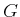is both a differentiable manifold and a group with respect to some operation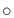if and only if:
1. The product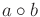, interpreted as a function from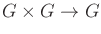, is smooth.
2. The inverse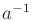, interpreted as a function fromto, is smooth.
The two conditions are needed to prevent the group from destroying the nice properties that come with the smooth manifold. An important result in the study of Lie groups is that all compact finite-dimensional Lie groups can be represented as matrix groups.

For any Lie group, a Lie algebra can be defined on a special set of vector fields. These are defined using the left translation mapping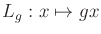. The vector field formed from the differential of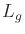is called a left-invariant vector field. A Lie algebra can be defined on the set of these fields. The Lie bracket definition depends on the particular group. For the case of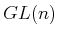, the Lie bracket is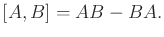(15.100)

In this case, the Lie bracket clearly appears to be a test for commutativity. If the matrices commute with respect to multiplication, then the Lie bracket is zero. The Lie brackets forandare given in many texts on mechanics and control [156,846]. The Lie algebra of left-invariant vector fields is an important structure in the study of nonlinear systems and mechanics.Steven M LaValle 2020-08-14